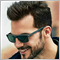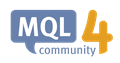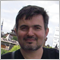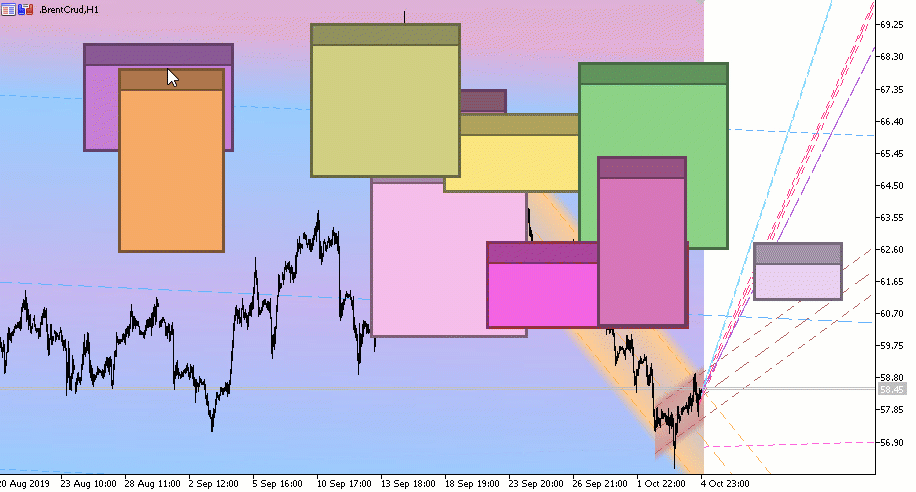how to convert all objects into 1 object94

Hi friends,

I created a Trade Panel using graphical library located in Inlcude/Controls.

need to know is it possible to convert all of these objects to only 1 object.

As you can see there are many objects in my Object List. need to have all of them in one object. Is it possible ?19843

1. Perhaps you can use canvas but you will have to code all object types yourself. Do you know how to draw a angled line on to a bit map?
2. Easier is to just set each object's OBJPROP_HIDDEN
Object Properties - Objects Constants - Constants, Enumerations and Structures - MQL4 Reference94

William Roeder:
1. Perhaps you can use canvas but you will have to code all object types yourself. Do you know how to draw a angled line on to a bit map?
2. Easier is to just set each object's OBJPROP_HIDDEN
Object Properties - Objects Constants - Constants, Enumerations and Structures - MQL4 Reference

I know how to hidden objects but need to all of these objects in one object.

Is is possible to use OBJ_RECTANGLE_LABEL for interface. and then convert all of objects on them to one object?OBJ_RECTANGLE_LABEL - Object Types - Objects Constants - Constants, Enumerations and Structures - MQL4 Reference
• docs.mql4.com
The function is used for creating and designing the graphical user interface. The frame type for the rectangular label can be selected from the enumeration ENUM_BORDER_TYPE. The following script creates and moves Rectangle Label object on the chart. Special functions have been developed to create and change graphical object's properties. You...19843

Martin Moreno: need to know is it possible to convert all of these objects to only 1 object.

Asked and previously answered in #1.1. There is no convert. You have to draw the pixels yourself — thousand+ lines of code.6362

Martin Moreno:

I know how to hidden objects but need to all of these objects in one object.

Is is possible to use OBJ_RECTANGLE_LABEL for interface. and then convert all of objects on them to one object?

Indeed, Canvas is the solution.

And Canvas is much easier than it seems at first glance.

Here is a primitive example of an indicator (MQL5 & MQL4) with one object OBJ_BITMAP_LABEL in which there are many windows.#property indicator_chart_window
#include <Canvas\iCanvas.mqh> //https://www.mql5.com/ru/code/22164 - MQL5
//https://www.mql5.com/en/code/23840 - MQL4

struct win {
int               x;
int               y;
int               width;
int               height;
uint              clr;
};
win wnd;
int OnInit() {
for (int i=0; i<ArraySize(wnd); i++) {
wnd[i].width=rand()%200+70;
wnd[i].height=rand()%150+50;
wnd[i].x=rand()%(W.Width-wnd[i].width);
wnd[i].y=rand()%(W.Height-wnd[i].height);
wnd[i].clr=ARGB(255,rand()%150+100,rand()%150+100,rand()%150+100);
}
ShowAllWind();
return(INIT_SUCCEEDED);
}

//+------------------------------------------------------------------+

int OnCalculate(const int rates_total, const int prev_calculated, const int begin, const double &price[]) {
return(rates_total);
}

//+------------------------------------------------------------------+

void OnChartEvent(const int id,
const long &lparam,
const double &dparam,
const string &sparam) {
static bool click = false;
static int x_mouse=0, y_mouse=0;
static int focus=-1, xfocus=0, yfocus=0;
int x=(int)lparam;
int y=(int)dparam;
if (sparam!="1" && click) focus=-1;
if (sparam=="1" && !click) {
focus=-1;
for (int i=ArraySize(wnd)-1; i>=0; i--) {
if (wnd[i].x<x && wnd[i].y<y && wnd[i].x+wnd[i].width>x && wnd[i].y+20>y) {
focus=i;
xfocus=x;
yfocus=y;
break;
}
}
if (focus>=0) ChartSetInteger(0,CHART_MOUSE_SCROLL,false);
else ChartSetInteger(0,CHART_MOUSE_SCROLL,true);
}
click=(sparam=="1")?true:false;
if (id==CHARTEVENT_MOUSE_MOVE && focus>=0) {
wnd[focus].x+=x-xfocus;
wnd[focus].y+=y-yfocus;
xfocus=x;
yfocus=y;
ShowAllWind();
}
if (id==CHARTEVENT_CHART_CHANGE) ShowAllWind();
}

//+------------------------------------------------------------------+

void ShowAllWind() {
Canvas.Erase();
for (int i=0; i<ArraySize(wnd); i++) {
Canvas.FillRectangle(wnd[i].x,wnd[i].y,wnd[i].x+wnd[i].width,wnd[i].y+wnd[i].height,ARGB(255,GETRGBR(wnd[i].clr)*0.5,GETRGBG(wnd[i].clr)*0.5,GETRGBB(wnd[i].clr)*0.5));
Canvas.FillRectangle(wnd[i].x+3,wnd[i].y+23,wnd[i].x+wnd[i].width-3,wnd[i].y+wnd[i].height-3,wnd[i].clr);
Canvas.FillRectangle(wnd[i].x+3,wnd[i].y+3,wnd[i].x+wnd[i].width-3,wnd[i].y+20,ARGB(255,GETRGBR(wnd[i].clr)*0.7,GETRGBG(wnd[i].clr)*0.7,GETRGBB(wnd[i].clr)*0.7));
}
Canvas.Update();
}
//+------------------------------------------------------------------+94

Nikolai Semko:

Indeed, Canvas is the solution.

And Canvas is much easier than it seems at first glance.

Here is a primitive example of an indicator (MQL5 & MQL4) with one object OBJ_BITMAP_LABEL in which there are many windows.

Thanks a lot dear Nikolai.